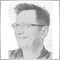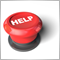new indicator debugging - page 22132

The current bar is always the one "receiving the ticks" (for minute bars from hh:mm:00 to hh:mm:59, for 10min bars from hh:m0:00 to hh:m9:59 and so on).

So the previous bar is last completed bar.

The price is an array of prices, the type ( open, close, mean and so on) you choose on the indicator setup.

So if you choose "open" you will always receive the opening price of the current bar, if you choose "close" you will receive always the last tick as the price.

Include the code in an indicator and "experiment" yourself.

int OnCalculate(const int rates_total,
const int prev_calculated,
const int begin,
const double &price[])
{
int bar_count = rates_total-prev_calculated;
if (bar_count==1) // the new bar arrived
{
Print(TimeCurrent()," last=",price[rates_total-2]," current=",price[rates_total-1]);
} else if (bar_count==0) // the new tick arrived
{
Print(TimeCurrent()," curr=",price[rates_total-1]);
} else {  // process history here
Print(TimeCurrent()," First Call");
}
return(rates_total);
}

Regards UweDocumentation on MQL5: Standard Constants, Enumerations and Structures / Indicator Constants / Price Constants
• www.mql5.com
Standard Constants, Enumerations and Structures / Indicator Constants / Price Constants - Documentation on MQL5218

angevoyageur:

The price is not a problem, the array provided with OnCalculate gives you price for each bar.

As I explained in my previous, and I can't be more clear than that, you have rewrite your calculations. Do you understood what I explained in this post ? You don't have to save all ema values, but at least this of the last bar.

I think I understood it partly, now the indicator works but I think i did not solved the problem I just bypassed it.

the problem for  me is: I don't know in the code how to know when the onCalculate starts to be called on new realtime (onTick) data and when instead is called on each bar on historical data.

so I used this very bad patch:

if(prev_calculated<rates_total) {
for(i=start;i<rates_total;i++) {
a = 2.0/(i+1.0);
ema = ema*oalpha+price[i]*alpha;
aux = ema-price[i];
emvar = emvar*oalpha + alpha*aux*aux;
EMSIGMABuffer[i] = MathSqrt(emvar);
emaBuff = emaBuff*(1.0-a) + EMSIGMABuffer[i]*a;
avgEMSIGMABuffer[i] = emaBuff;
}
}

by the way I red that your job is to make mql5 programs upon request, how much is your rate? and do you teach as well? in case you do how much is your rate?39026

michelino:

I think I understood it partly, now the indicator works but I think i did not solved the problem I just bypassed it.

the problem for  me is: I don't know in the code how to know when the onCalculate starts to be called on new realtime (onTick) data and when instead is called on each bar on historical data.

so I used this very bad patch:

by the way I red that your job is to make mql5 programs upon request, how much is your rate? and do you teach as well? in case you do how much is your rate?

See your a PM.218

angevoyageur:
See your a PM.
sorry. what does it mean?39026

michelino:
sorry. what does it mean?
PM= private message.MQL5.community - User Memo
• 2010.02.25
• MetaQuotes Software Corp.
• www.mql5.com
You have just registered and most likely you have questions such as, "How do I insert a picture to my a message?" "How do I format my MQL5 source code?" "Where are my personal messages kept?" You may have many other questions. In this article, we have prepared some hands-on tips that will help you get accustomed in MQL5.community and take full advantage of its available features.39026

Anyway I made the following changes :

if(prev_calculated==0)
{
start=period-1+begin;
ema=price[begin];                 //start+1-period
emvar=0.0;
EMSIGMABuffer=0.0;
avgEMSIGMABuffer=0.0;
emaBuff=emvar;
} else {
start=prev_calculated-1;
ema=price[prev_calculated-period];
emvar=MathPow(EMSIGMABuffer[start-1],2);
emaBuff=avgEMSIGMABuffer[start-1];
}

You have to check if that works.218

angevoyageur:

Anyway I made the following changes :

You have to check if that works.

it works. thanks a lot